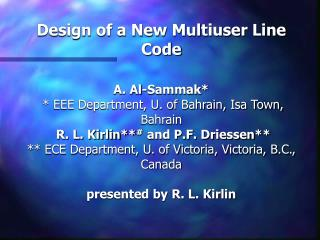DownloadDownload PresentationObjectives

# Objectives

Télécharger la présentation## Objectives

- - - - - - - - - - - - - - - - - - - - - - - - - - - E N D - - - - - - - - - - - - - - - - - - - - - - - - - - -
##### Presentation Transcript

1. Design of a New Multiuser Line CodeA. Al-Sammak** EEE Department, U. of Bahrain, Isa Town, BahrainR. L. Kirlin**# and P.F. Driessen**** ECE Department, U. of Victoria, Victoria, B.C., Canadapresented by R. L. Kirlin

2. Objectives • Find a code that allows multiple users to simultaneous access the same data channel • Maximize efficiency, trading off • number of users • number of symbols per user • number of bits per symbol • Compare to other schemes

3. Results • May use as a single user line codeby partitioning data stream into “users’” symbols. • Produces higher rates than QAM and TDM because higher dimensional signal space is used • Up to 4.4 bits/baud for 22 users, 2 symbols/user, and 5 bits/symbol

4. Methodology • Similar to collaborative coding multiple access (CCMA) techniques • Permit simultaneous transmission by multiple users over a common binary adder channel or over a shared radio channel • Combined users’ symbol codes must be uniquely decodeable

5. Example coding process U = 3 users’ source symbols, M = 2 symbols/user, assigned to 2-bit words, m (only for cc) B = 2-time-slot data word V transmitted 1 0 1 0 1 0 1 1 1 Lookup table combines mi 1 3

6. Problems • What is the maximum number of users U each choosing from M symbols, that can use B bit transmission words? • Alternately, given U users, what is the minimum number B of bits that is required. • How do we decode the M user symbols associated with each V?

7. EXAMPLE • Consider the U = 4-users, M = 2 symbols/users, B = 2-bits/symbol code. • Needs only MU = 24 = 16 points from the Nv = (U+1)B = 25 possible combinations V in the 2-dimensional space, i.e. from the grid of size (U+1)B = 52 25 points ...

8. Example showing size and use of signal space; U = 4, M = 2, B = 2 t2 t2 t1 t1 U=4, All (U+1)B = 25 possible points U=4, MU = 16 points selected for transmission

9. We may use “QAM-like” constellations, as shown for the U = 4 users B = 2 time slots

10. Alternate constellation diagram for U=4 , B=2, M = 2 symbols/user illustrating both direct transmit level coding and PAM transmission.

11. Table 1: Constellations for 4-user code Constellation user symbols Direct Tx Symmetric assignment u1 u2 u3 u4 levels PAM 1 0000 2 1 0 –1 2 0001 0 1 -2 –1 3 0010 1 0 -1 –2 4 0011 0 0 -2 –2 5 0100 3 2 1 0 6 0101 4 1 2 –1 7 0110 3 0 1 –2 8 0111 4 0 2 –2 9 1000 1 2 -1 0 10 1001 0 3 -2 1 11 1010 1 4 -1 2 12 1011 0 4 -2 2 13 1100 2 3 0 1 14 1101 4 3 2 1 15 1110 3 4 1 2 16 1111 4 4 2 2

12. Implications • The number of dimensions in the signal space is B. • The number of possible unique values (levels) of the sum vectors V is NVmax = (U+1)B. • The maximum number of unique symbols available to all users is Mmax = 2B. • The maximum number Nw of symbol combinations is MmaxU. • Because the actual number Nw words used must be less than or equal to NVmax , we have the constraint: • Nw = MU  NVmax = (U+1)B

13. A most important point : We can use the constraint two ways: either 1) For fixed U and B we can select an M small enough that MU (U+1)B or 2) For a fixed M and U we may increase B so that MU  (U+1)B.

14. Information Rate is defined as • R = log2 M * (U/B) • user information bits / time-slot • Code Efficiency is defined as the symbol combinations used by the U users divided by the number of symbols available given B-bit symbols, •  = MU/(U+1)B  1

15. Conclusions • The code may be used as a single user line codeby partitioning data stream into blocks of U “users’” symbols. • U users’ simultaneous source symbols are encoded by lookup table according to the design, and decoded similarly. • It produces higher bits/baud or bits/dimension than QAM and TDM because a higher dimensional signal space is used (B bits / symbol).

16. Maximum M , symbols/user and maximum number of users U, have been tabulated for most realistic cases when other parameters are fixed. • Up to 4.4 bits/baud (bits/time slot) results with U = 22 users, M = 2 symbols/user, and B = 5 bits/symbol. • Design objectives have been achieved